# 详解最大似然估计（MLE）、最大后验概率估计（MAP），以及贝叶斯公式的理解

## 贝叶斯公式到底在说什么？

P(A|B)=P(B|A)P(A)P(B) $P(A|B) = \frac{P(B|A)P(A)}{P(B)}$ 【式1】

P(A|B)=P(B|A)P(A)P(B|A)P(A)+P(B|A)P(A) $P(A|B) = \frac{P(B|A)P(A)}{P(B|A)P(A) + P(B|\sim A)P(\sim A)}$ 【式2】（ A $\sim A$表示”非A”）

——————不行，还得先说似然函数（likelihood function）

## 似然函数

P(x|θ) $P(x|\theta)$

## 最大似然估计（MLE）

f(x0,θ)=(1θ)×θ×θ×θ×θ×(1θ)×θ×θ×θ×(1θ)=θ7(1θ)3=f(θ) $f(x_0 ,\theta) = (1-\theta)\times\theta\times\theta\times\theta\times\theta\times(1-\theta)\times\theta\times\theta\times\theta\times(1-\theta) = \theta ^ 7(1 - \theta)^3 = f(\theta)$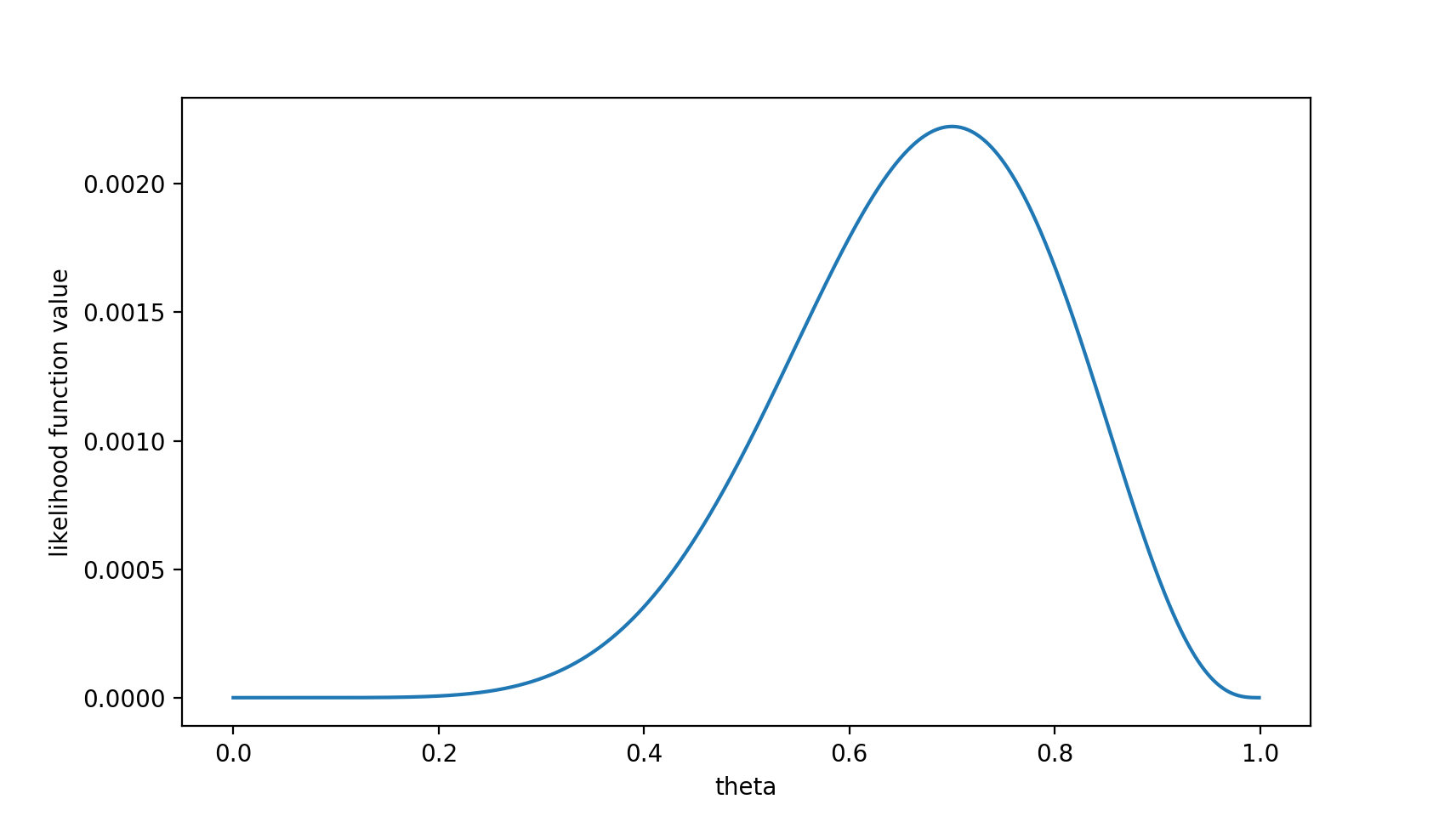## 最大后验概率估计

MAP其实是在最大化 P(θ|x0)=P(x0|θ)P(θ)P(x0) $P(\theta|x_0) = \frac{P(x_0|\theta)P(\theta)}{P(x_0)}$，不过因为 x0 $x_0$是确定的（即投出的“反正正正正反正正正反”）， P(x0) $P(x_0)$是一个已知值，所以去掉了分母 P(x0) $P(x_0)$（假设“投10次硬币”是一次实验，实验做了1000次，“反正正正正反正正正反”出现了n次，则 P(x0)=n/1000 $P(x_0) = n/1000$。总之，这是一个可以由数据集得到的值）。最大化 P(θ|x0) $P(\theta | x_0)$的意义也很明确， x0 $x_0$已经出现了，要求 θ $\theta$取什么值使 P(θ|x0) $P(\theta | x_0)$最大。顺带一提， P(θ|x0) $P(\theta | x_0)$即后验概率，这就是“最大后验概率估计”名字的由来。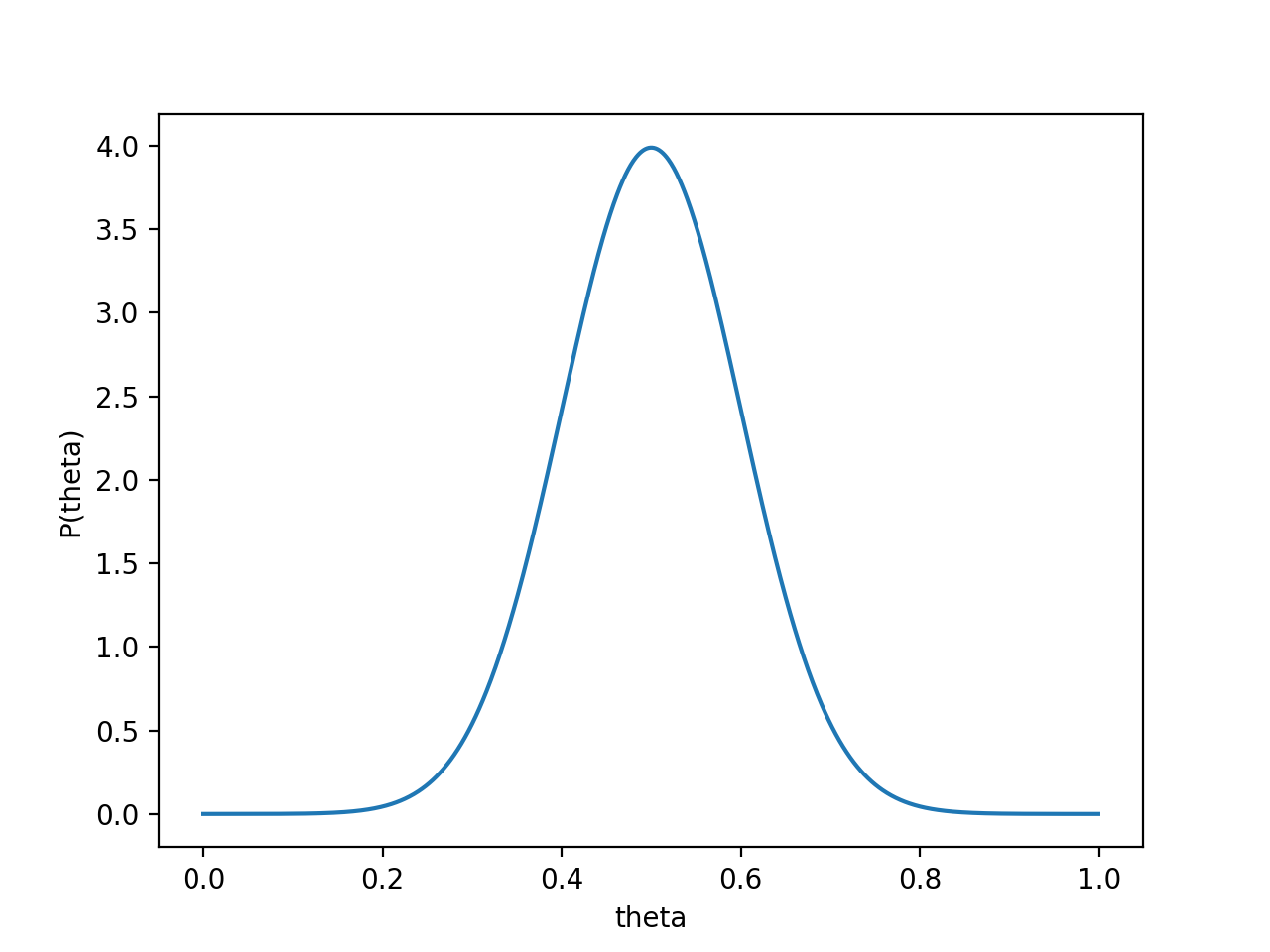P(x0|θ)P(θ) $P(x_0 | \theta) P(\theta)$的函数图像为：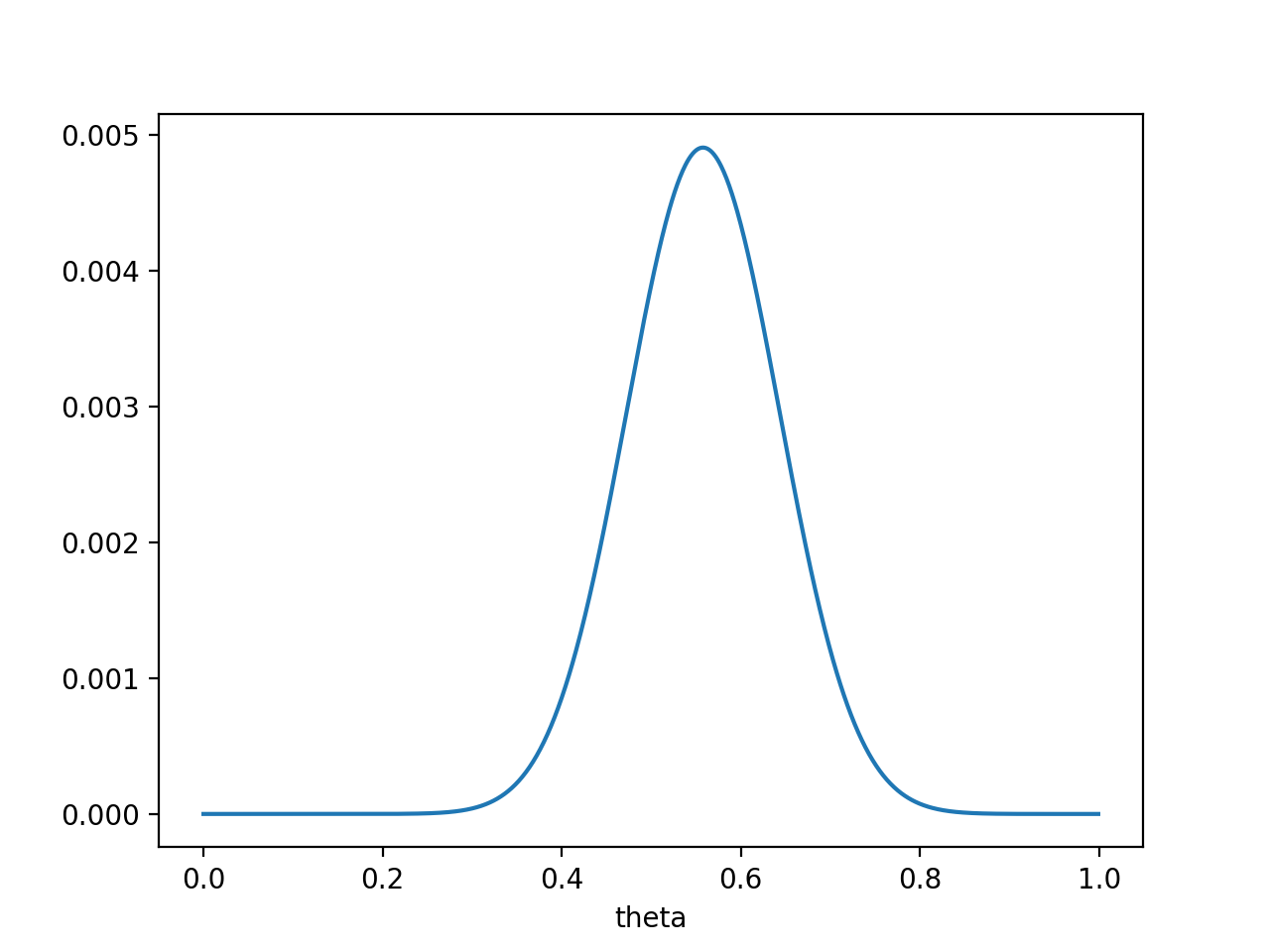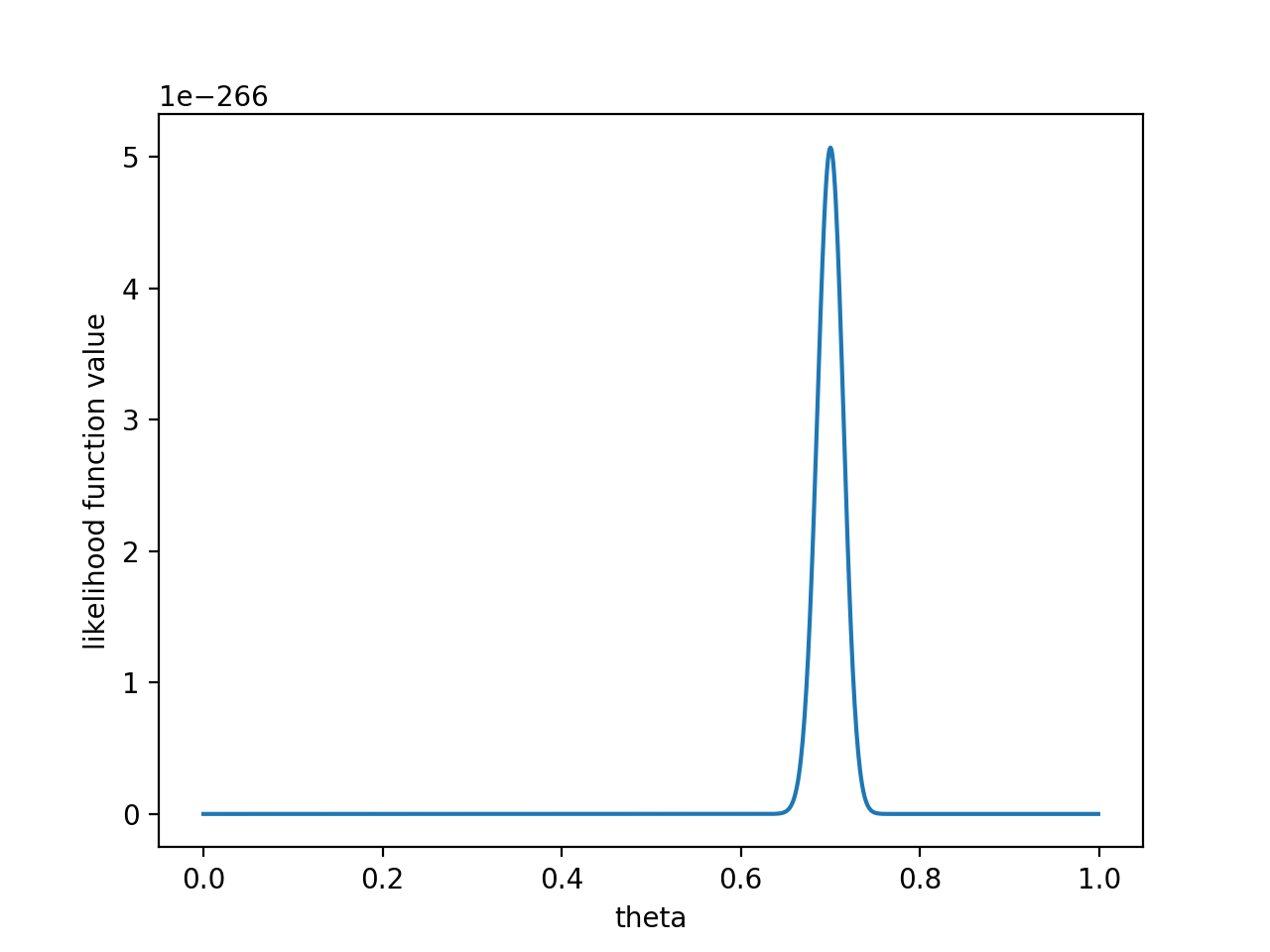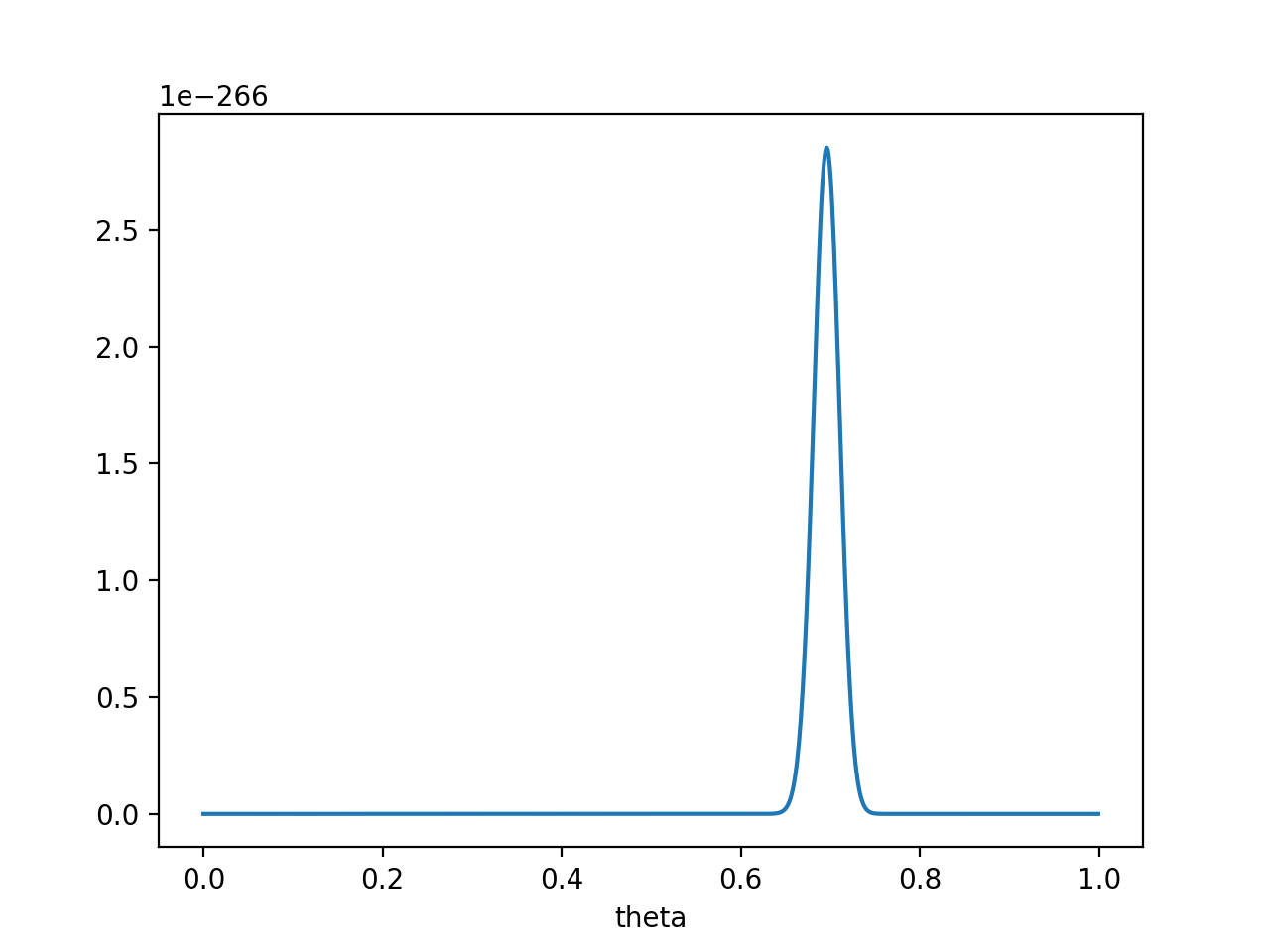θ=0.696 $\theta = 0.696$处， P(x0|θ)P(θ) $P(x_0 | \theta) P(\theta)$取得最大值。

PS. 要是遇上了顽固的贝叶斯派，认为 P(θ=0.5)=1 $P(\theta = 0.5) = 1$ ，那就没得玩了。。 无论怎么做实验，使用MAP估计出来都是 θ=0.5 $\theta = 0.5$。这也说明，一个合理的先验概率假设是很重要的。（通常，先验概率能从数据中直接分析得到）

## 最大似然估计和最大后验概率估计的区别

12-071万+
06-13931208-058万+
05-2824万+
01-20393
08-071万+
03-261万+
12-271374
10-055890
09-27556